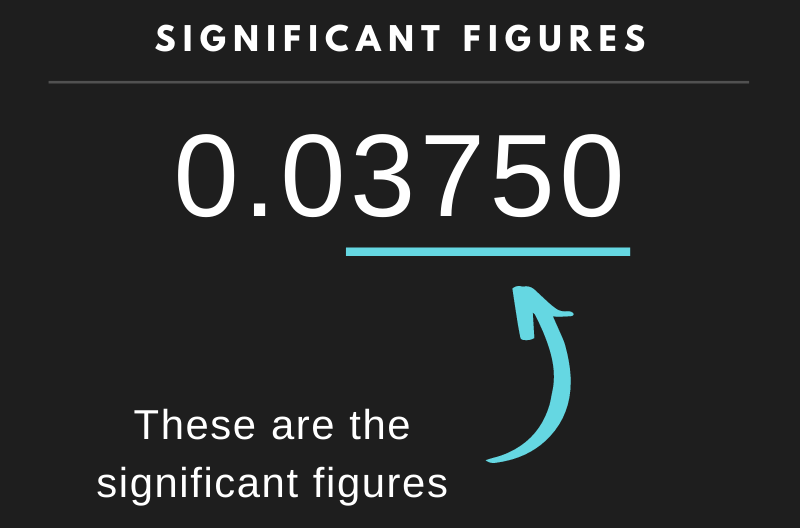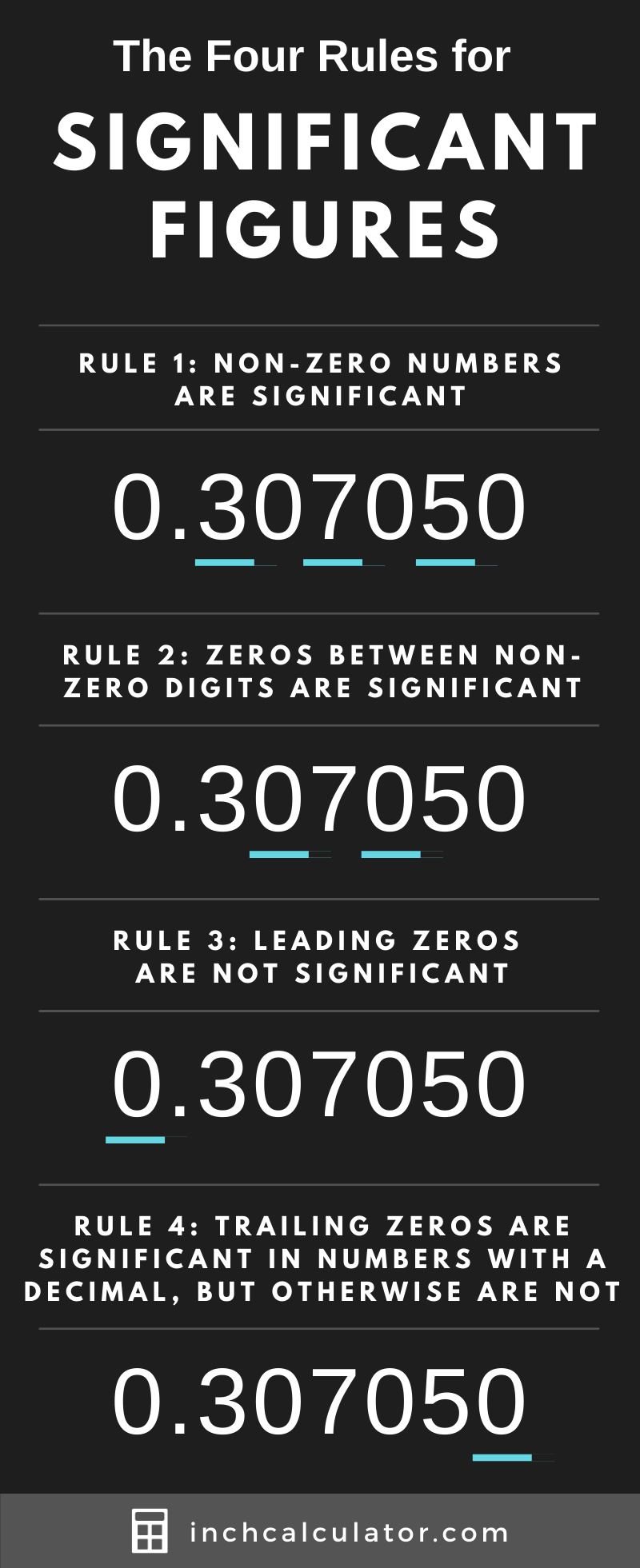# Sig Fig Calculator (Significant Figures)

Calculate the significant figures in a number or round a number using the sig fig calculator below. For numbers in scientific notation, use e notation (e.g. 3.1415e5).

## Count of Significant Figures:

### The Significant Figures Are:

Learn how we calculated this below

## What are Significant Figures?

The significant figures of a number, also referred to as its significant digits, are the digits in a number that are meaningful in expressing its precision. In other words, these are the digits that provide meaning to the overall number.

Significant figures are most commonly used when making measurements and are the important digits that tell us something about the precision of the number or measurement, and are often used to simplify or round a number without losing that precision.

Significant figures do not quantify the size of a number but rather the level of precision, which is useful in converting from decimal to scientific notation or standard form.

## How to Find Significant Figures

Since not all digits in a number are significant, there are a series of rules to follow to find which are the significant figures.

### Significant Figures Rules

The following are the rules for finding significant figures:

• All non-zero numbers ARE significant. There are three digits in the number 3.14 that are significant because each digit is non-zero.
• Zeros between non-zero digits ARE significant. The zero in the number 4.605 is significant because it’s between two non-zero numbers.
• Leading zeros ARE NOT significant. Any zero to the left of non-zero digits ARE NOT significant. The zero in the number 0.47 is not significant.
• Trailing zeros in a number with a decimal point ARE significant, but trailing zeros in a number without a decimal point ARE NOT significant. The zero in the number 470 is not significant, but the zeros in 470.0 are significant.When a number has a decimal point but no trailing digits, the decimal point indicates that all the digits in the number are significant. For instance, the number 750 has precision to the one’s place and thus has three significant digits.

You can use an overline to indicate the last significant figure in a number. However, some people choose to use an underline in place of an overline.

### Examples

Table showing the significant figures for various numbers
Count of Significant Figures Significant Figures
23 2 2, 3
7.5 2 7, 5
0.00025 2 2, 5
10.7 3 1, 0, 7
-12.208 5 1, 2, 2, 0, 8
63500 3 6, 3, 5
63500. 5 6, 3, 5, 0, 0
63500.0 6 6, 3, 5, 0, 0, 0

## How to Round Significant Figures

It is common to round a number to a specified number of significant figures, and the process is similar to rounding a decimal. Follow these steps to round a number with significant figures found using the sig fig rules above.

### Step One: Find Significant Figures

The first step to round a number to a sig fig is to find the significant digits in a number. Follow the rules above to find the figures that are significant, then move to the next step.

### Step Two: Use Rounding Rules

Once you’ve found the significant figures, use standard rounding rules to round the number to the specified precision. The difference between sig fig rounding and standard decimal rounding is that the rounding point is the significant digit indicated by the precision rather than the decimal place.

### Putting it all Together

Let’s follow the steps above to round 03570 to two significant figures.

The number 03540 has three significant digits: [3, 5, 7]

Round the number to the position of the second sig fig, which is 5:

03570 -> 3600

### Sig Fig Rounding Examples

Table showing a number rounded to various significant figures
Sig Fig Precision Rounded to Significant Figures
375.09 0
375.09 1 400
375.09 2 380
375.09 3 375
375.09 4 375.1
375.09 5 375.09
375.09 6 375.090
375.09 10 375.0900000

### Why do we use significant figures?

We use significant figures to express the precision of a number or, more specifically, of a measurement. For example, if we measure the length of a box with a ruler and the smallest ticks on the ruler are the centimeter ticks, then we only have precision down to the number of centimeters.

Therefore, the number of centimeters measured would be significant digits, but not any fraction of a centimeter since we only have precision to whole centimeters.

### Are exact numbers significant figures?

Yes, exact numbers have all significant figures, following the rules listed above.

### Are significant figures the same as significant digits?

Yes, significant digits is another term often used interchangeably with significant figures.

### Are numbers with more significant figures more accurate?

This is a common misconception. Numbers with more significant figures are not necessarily more accurate, but they are certainly more precise. Accuracy and precision are two distinct concepts.

## Visual Guide to Significant Figures

The infographic below demonstrates the four rules for significant figures.## References

1. Columbia Center for Teaching and Learning, Significant Figures, https://ccnmtl.columbia.edu/projects/mmt/frontiers/web/chapter_5/6665.html